We use cookies to provide you with a great user experience. By using our site, you accept our use of cookies. You can review our cookie and privacy policy here.
• Support

Our Customer Service team is available from 8am to 6:00pm, ET, Monday through Friday. Live chat is available from 8am to 5:30pm ET, Monday-Friday.

Call:
800.334.5551
Fax:
Email:
Email Customer Service

• Cart
0

### My Cart

Your Shopping Cart is currently empty. Use Quick Order or Search to quickly add items to your order!

# Summary of the Equation for the Magnitude of Centripetal ForceFelicia Cherry
Product Manager for Physical Science, Physics, and Earth Science

Almost every physics class covers circular motion. Students spend considerable time learning the basic formula and solving problems. This article describes an algebra-based derivation of the equation for centripetal force.

### Deriving the equation

This first equation should be familiar. It is probably the most used equation in any first-year physics course and the way most physics students learn to express Newton’s Second Law: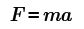Equation 1.

The net force on an object is equal to the mass of the object times the acceleration of the object. Equation 2 applies this principle to objects moving in uniform circular motion, where the object moves at a constant speed but continues to change direction so that it travels in a circular path. An object moving in uniform circular motion is acted on by a force directed at the center of the circular path, called a centripetal force. Equation 2 restates Equation 1 for objects moving in uniform circular motion. Fc stands for centripetal force, and ac stands for centripetal acceleration.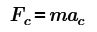Equation 2.

The equation for the magnitude of centripetal force is given by: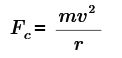Equation 3.

When describing linear motion, acceleration is usually described as the change in velocity divided by the change in time. Mathematically, acceleration may be expressed like this: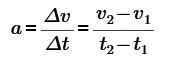Equation 4.

From Equation 2 and Equation 3, centripetal acceleration, or ac, can be expressed as: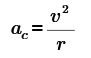Equation 5.

The next section will explain why centripetal acceleration is equal to velocity squared divided by the radius, as written in Equation 5.

The following list defines the symbols used in this section:
F = the magnitude of the force (in newtons, N)
Fc = the centripetal force, directed at the center of the circular path (N)
m = the mass of the object traveling in a circular path (in kilograms, kg)
v = the tangential velocity of the object (meters per second, m/s)
v1 = initial velocity (meters per second, m/s) (in some texts this is vi or v0)
v2 = final velocity (meters per second, m/s) (in some texts this is vf)
r = the radius of the circular path (in meters, m)
a = the magnitude of the acceleration (in meters per second squared, m/s2)
ac = the centripetal acceleration, directed at the center of the circular path (m/s2)
Δ indicates change, for example Δv = (v2v1)

Note: This derivation analyzes the vectors describing the motion of an object moving in uniform circular motion and uses similar triangles to relate the velocity of the object with the radius of the circular path.

### Why centripetal acceleration is equal to velocity squared divided by the radius

Consider an object moving at a constant speed in a circular path. The object’s speed is constant, but the velocity changes because the direction of the object is constantly changing. Consider the velocity at two moments during the circular path. In Fig. 1, v1 represents the initial velocity. In Fig. 2, v2 represents the velocity after a short time interval.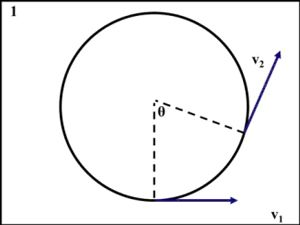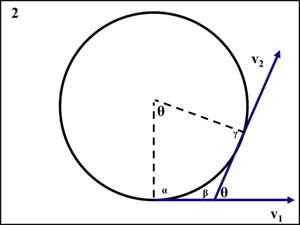Equation 1.

Figure 1. Diagrams 1 (left) and 2 (right) illustrate the change in direction of the velocity vector from time 1 to time 2. Diagram 2 illustrates that the angle between the velocity vectors is equal to the angle between the radii at time 1 and time 2.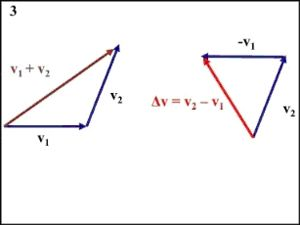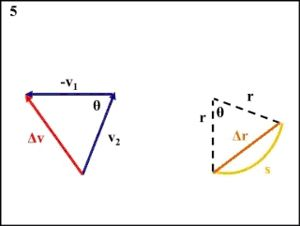Figure 2. Diagrams 3, 4, and 5.

As long as the length and direction of the vectors remain unchanged, they can be drawn any way that is convenient. Diagram 3 illustrates adding and subtracting vectors v1 and v2.

The vectors -v1, v2, and Δv form a triangle that is similar to the triangle formed by the radii drawn at time 1 and time 2, and the chord Δr.

The magnitude of vectors v1 and v2 is equal and has a magnitude of v.

v1│ = │v2│ = v

Because the triangles are similar, the following is true: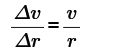Equation 6.

Δr = the length of the chord between the location of the object at time 1 and time 2.
r = the radius of the circle.

The circular segment s represents the distance traveled by the object from time 1 to time 2.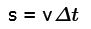Equation 7.

If the angle θ is very small, the following approximation can be made.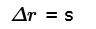Equation 8.

This allows the following substitution: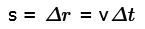Equation 9.

Substituting vΔt for Δr into Equation 6: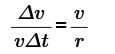Equation 10.

Rearranging for Δv/Δt gives Equation 11.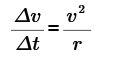Equation 11.

Δv/Δt is acceleration as seen in Equation 4: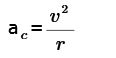Multiplying the acceleration by the mass of the object gives the centripetal force as seen in Equation 3.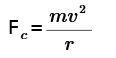Equation 3 and Equation 4 can be used to solve many physics problems.

### Resources

The equations for centripetal force and centripetal acceleration may be applied to these products.